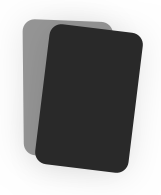# MATH 117 test 1

0.0(0) Reviews
Duplicate
Report Flashcard set

#### Spaced Repetition

Scientifically backed study method#### Flashcards

Review terms and definitions#### Learn

Study with MC, T/F, and other questions#### Practice Test

Take a test on your terms and definitions35 Terms
😃 Not studied yet (35)
Set
is a collection of distinct "things".
Cardinality
is the number of things in a set
Place Value
the quantity that a digit in a number represents depends - in a very specific way- on the position of the digit in the number
Expanded form
shows how it is composed of its place value parts. ex, 536 is 5 hundred 3 tens 6 ones
Number line
is a line on which we have chosen one location as 0 and another location as 1.
Base ten
the essence of the base ten systems was that every time we move to the left, we multiply by 10
Scale
the choice of a unit
Negative number
-N as the "opposite" of N
Absolute value
is the distance of M (a number) from 0.
Explain greater than and less than numbers
- look at the place value of the digits - determine if the number is positive or negative - the number line explanation
explain how to round
if the digit to the right of the place value we are being asked to round is 5 or greater then we round the digit and make every number after it 0.
Multiple
numbers that are the product of counting numbers
Factors
a number that divides another number evenly with no remainder
Even number
a number that is divisible by 2 with no remainder
Odd number
a number that is not evenly divisible by 2
quotient
the answer you get from dividing two numbers
divisor
the number you divide by
Divisibility test
a method for checking to see if a counting number is divisible by another counting number without actually carrying out the division.
divisibility test for 2
If the number is even, yes. if the number is odd, no
divisibility test for 10
if the ones digit is 0, yes. if the ones digit is not 0, no.
divisibility test for 5
if ones digit is 0 or a 5, yes. if ones digit is not a 0 or a 5, no
divisibility test for 3
add the digits of the number. if the sum of the digits is divisible by 3, yes
divisibility test for 9
add the digits of the number. if the sum is divisible by 9, yes.
divisibility test for 4
only consider the last two digits. if the number formed by the last two digits is divisible by 4 then yes.
prime numbers
are the counting numbers that are divisible by only 1 and themselves.
what are the first few prime numbers?
2,3,5,7,11,13,17, 19,23,29,31, and 37
composite numbers
are counting numbers that are not prime.
how to find the list of primes?
Sieve of Eratosthenes - start with 2 and cross out every 2nd number, then do 3 and every 3rd number, then 5, and every 5th number ....
Trial division
division by prime numbers to find out if the given number is prime. stop when your quotient (your answer) is smaller than the divisor (the number you divide by) .
factor tree
is a diagram that shows how to factor a number until the prime numbers are reached.
greatest common factor
- is the greatest counting number that is a factor of all the given counting numbers
least common multiple
is the smallest counting number that is a multiple of all the given counting numbers
The slide method
- make a table with the two numbers in separate columns with one extra column in the front - write a common factor in the first column and the quotient (so your dividing) in the row beneath your initial numbers. - repeat this process until the only common factor you have is 1 GCF = multiply all the common factors (the number in the first column) LCM = multiply all the common factors and the last row of quotients
how to find the GCF of fractions
- find the greatest common factor of the top and bottom number of the fraction - divide the top and bottom numbers by the GCF - record results
how to find the LCM of fractions
- to add fractions look at the bottom of the fraction and find the least common multiple of the two numbers - then create new fractions with the LCM ( multiply the original fraction with the number you multiplied by to get the LCM.) - then add the product of both the fraction equations.